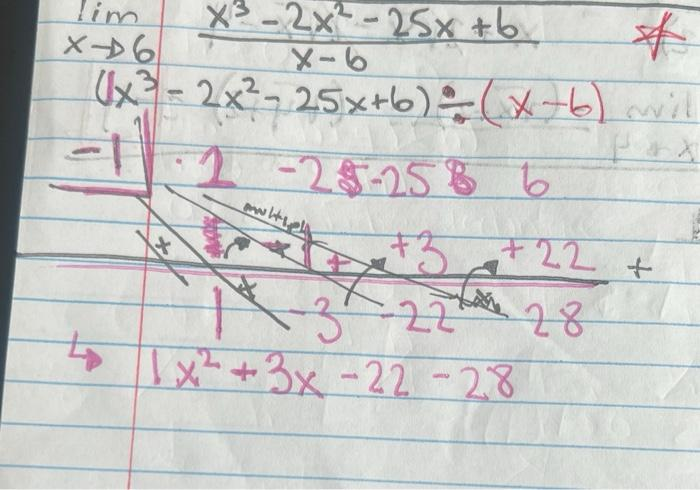Home / Expert Answers / Calculus / can-i-see-where-my-mistake-is-thanks-nbsp-lim-x-rightarrow-6-frac-x-3-2-x-2-25-x-6-x-pa560

# (Solved): can i see where my mistake is thanks  $$\lim _{x \rightarrow 6} \frac{x^{3}-2 x^{2}-25 x+6}{x- ... can i see where my mistake is thanks\( \lim _{x \rightarrow 6} \frac{x^{3}-2 x^{2}-25 x+6}{x-6}$$ $$\left(x^{3}-2 x^{2}-25 x+6\right) \div(x-6)$$ $$1 x^{2}+3 x-25-25+6$$

We have an Answer from Expert